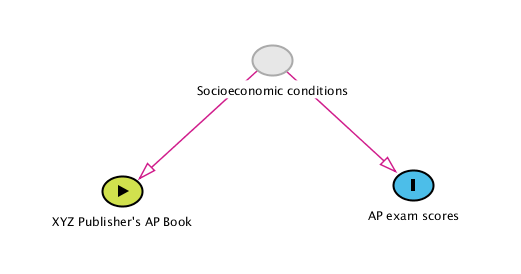## Introduction

Causal Inference, a branch of statistics, enables us to make quantitative and qualitative conclusions about the effect of a particular exposure or treatment on how the treatment alone affects a study subject’s results. The standard methods of causal inference uses the Stable Unit Treatment Value Assumption (SUTVA), which requires that the exposure of one individual not affect the outcome of another. The presence of interference - that is, the phenomenon when one person getting treated affects another person’s outcome - invalidates this assumption. This post investigates how we can adapt our repertoire of methods from Causal Inference to situations with interference.

## What is Causal Inference?

Say we want to find out how a particular treatment affects the outcome of a situation. This could be the result of a drug trial, or the effect of a policy on how it affected the public. We could try and find out how those who got treated differed from those who did not. This can be done by a simple linear regression of the form $$Y \sim A$$ and seeing if the coefficient on A is statistically significant. At this point one is reminded of the ubiquitous mantra “Correlation does not imply causation”. What if the treated population is just fundamentally different from those that were untreated?

We can express our dilemma using the figure below. What if instead of the treatment, $$A$$, affecting the outcome, $$Y$$, directly, there is some hidden variable that we do not account for in our analysis? In the situation represented by the graph below, XYZ publisher could claim that using their books leads to higher scores in AP exams by showing a statistical correlation, when the underlying reality is that students with higher family incomes tend to buy XYZ Publisher’s books, and they’re also the ones getting better AP scores on average.Causal Inference deals with this dilemma, of trying to find only how one particular variable (the treatment) affects the outcome in a situation of interest. We now introduce the conceptual framework for how a causal inference analysis is done.

For a particular subject in a study, assume we can either treat them, or not. Let the treatment be represented by $$A$$, with $$A=0$$ representing no treatment, and $$A=1$$ representing treatment. Then the person with have an outcome $$Y$$, which might or might not vary depending on $$A$$. In reality we only see $$Y|A=1$$ or $$Y|A=0$$. Let $$A = a$$, where $$a \in \{0,1\}$$. A variable which denotes the possible outcomes for both treatment values can then be represented as $$Y^a$$. We refer to $$Y^a$$ as the potential outcome.

Each person’s potential outcomes can have two values, the treated value ($$Y^{a=1}$$) and the untreated value ($$Y^{a=0}$$). We can find the causal effect of treatment on one individual by calculating $$Y^{a=1}-Y^{a=0}$$. We can average this over all individuals to find the average causal effect, that is, $ACE = E[Y^{a=1}]-E[Y^{a=0}]$

Note that of the two potential outcomes, only one is observed in reality. There are different ways in which we can find the value for the missing potential outcome . We subsequently discuss one particular method of achieving this using randomization of treatment, but there are other techniques such as Inverse Probability Weighting that can be used to this end (Hernan and Robins, 2020).

A crucial idea in causal inference is that of exchangeability. If the individuals in either of the treatment groups (control or treated) have the same characteristics, one would expect them to have the same outcomes if they get the same treatment. We say that exchangeability holds in this case. This can be expressed as $$Y^a \perp \!\!\! \perp A$$ - the actual distribution of potential outcomes for the population is independent of the treatment. As long as we can ensure exchangeability holds between treatment groups, we can use the actual observed outcomes instead of the potential outcomes, half of which are not observed in reality. When exchangeability holds, the average causal effect becomes $$E[Y|A=1]-E[Y|A=0]$$.

One way to achieve exchangeability is by randomizing the treatment assignment. Since treatments are randomly assigned, we are assured that on average, a similar population is represented in both the treated and control groups.

### Causal Inference Assumptions

In the above process for causal interference we must make the Stable Unit Treatment Value Assumption, or SUTVA (Rubin, 1980, as cited in Hernán and Robins, 2020). SUTVA has two requirements:

• The treatment value of one individual does not affect the potential outcome of another individual. In other words, there is no interference.

• There are not different types of exposure (for example, when two people both receive the treatment, it is not the case that one of them gets a medicine that is past the due date).

What happens if SUTVA does not hold? Say we have a setting like a vaccine study, where after a particular number of people get vaccinated, others are much less likely to get a communicable disease because of herd immunity. In this case the first assumption of SUTVA - that of no interference - does not hold. It turns out that potential outcomes are not binary in such a situation. This project investigates what strategies can be adopted to study causal effects in situations with interference.

## Causal Inference with Interference

As we defined earlier, interference is said to occur if the potential outcomes of one individual depend on the treatment value of another individual. The causal graphs below demonstrate a situation without interference and with interference.

First, we see a situation without interference. The effect of treatment on individual $$1$$, $$A_1$$, only affects the outcome of individual $$1$$. The same is true for individuals $$2$$ and $$3$$.